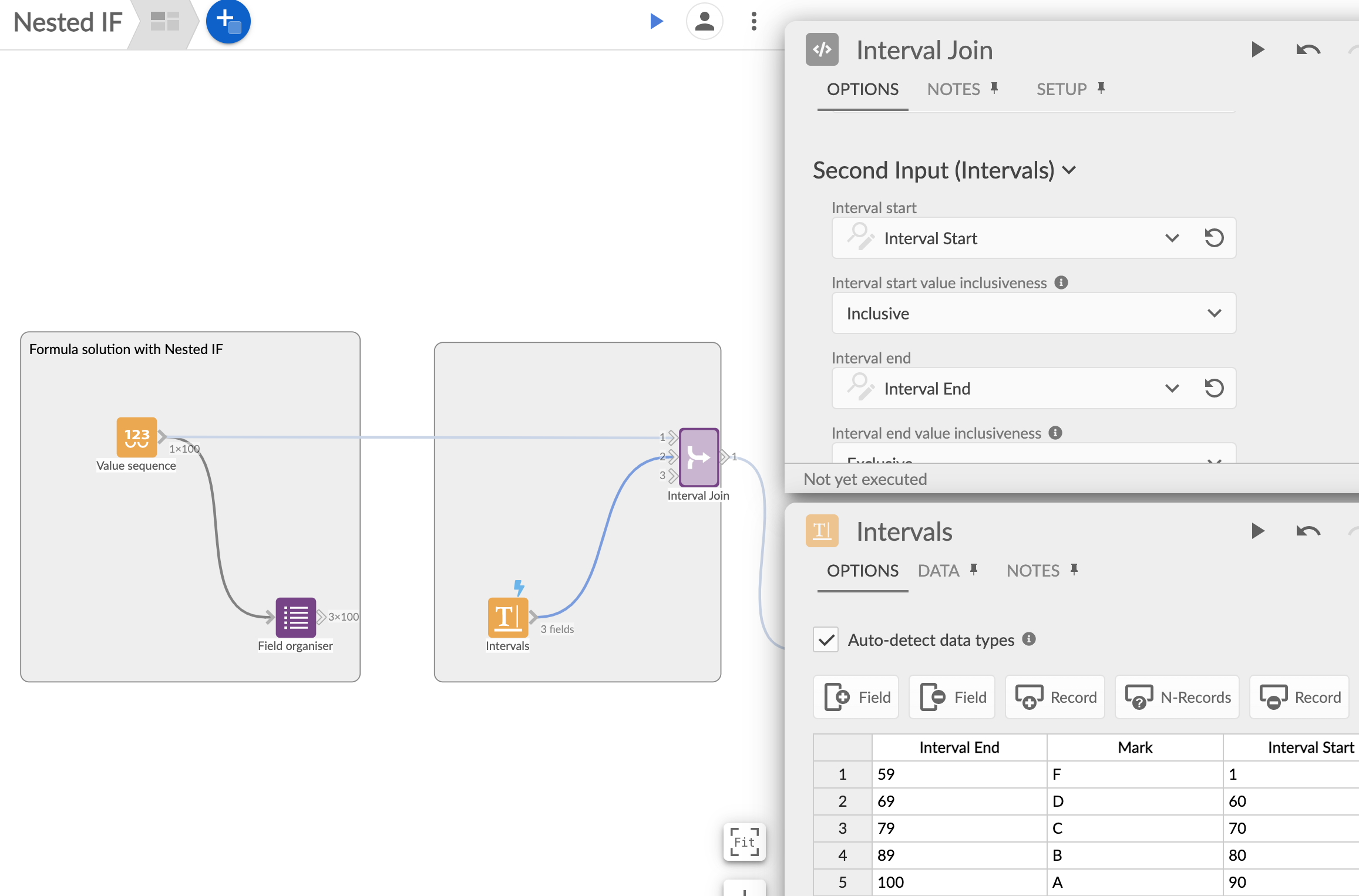## Nested IF formula + Interval Join block - two methods of creating data intervals and allocating values

There are several solutions for a scenario where the user needs to assign a value based on some other parameter e.g. exam marks allocation - depending on points, postage price based on parcel weight, commission or tax % based on revenue etc.

In Omniscope you can use a nested IF formula, where you're building from the smallest value up, or from the highest value down.

In an example where the student can score between 0 and 100 points on a test here are the mark allocation formulas.

Exam scoring - points Vs mark:

0-59 --> F

60-69 --> D

70-79 --> C

80-89 --> B

90-100 --> A

IF(

[Points]>89,"A",

[Points]>79,"B",

[Points]>69,"C",

[Points]>59,"D",

"F")

IF(

[Points]<=59,"F",

[Points]<=69,"D",

[Points]<=79,"C",

[Points]<=89,"B",

"A")

c) Alternatively there is an 'Inequality block', where the process can be automated and interval allocation easily updated.

Interval Join block requires an additional input file, which contains the boundaries and the allocated values.

Another requirement is an installation of the R package, as per Setup block's instructions.